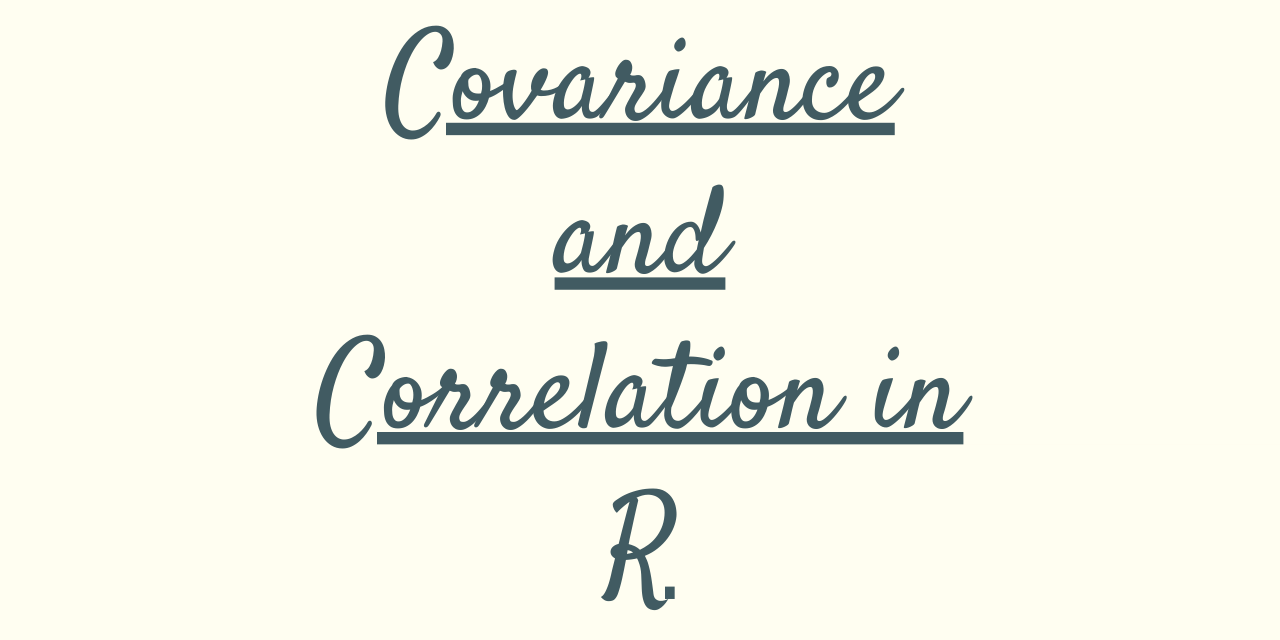# Covariance and Correlation in R programming

Published on August 3, 2022By Safa MulaniWhile we believe that this content benefits our community, we have not yet thoroughly reviewed it. If you have any suggestions for improvements, please let us know by clicking the “report an issue“ button at the bottom of the tutorial.

Hello readers! In this article, we would be focusing on two important parameters of statistics – Covariance and Correlation in R programming, in detail.

So, let us begin!!

## Covariance in R programming

In Statistics, Covariance is the measure of the relation between two variables of a dataset. That is, it depicts the way two variables are related to each other.

For an instance, when two variables are highly positively correlated, the variables move ahead in the same direction.

Covariance is useful in data pre-processing prior to modelling in the domain of data science and machine learning.

In R programming, we make use of `cov() function` to calculate the covariance between two data frames or vectors.

Example:

We provide the below three parameters to the cov() function–

• x – vector 1
• y – vector 2
• method – Any method to calculate the covariance such as Pearson, spearman. The default method is Pearson.
``````a <- c(2,4,6,8,10)

b <- c(1,11,3,33,5)

print(cov(a, b, method = "spearman"))

``````

Output:

``````> print(cov(a, b, method = "spearman"))
 1.25
``````

## Correlation in R programming

Correlation on a statistical basis is the method of finding the relationship between the variables in terms of the movement of the data. That is, it helps us analyze the effect of changes made in one variable over the other variable of the dataset.

When two variables are highly (positively) correlated, we say that the variables depict the same information and have the same effect on the other data variables of the dataset.

The `cor() function` in R enables us to calculate the correlation between the variables of the data set or vector.

Example:

``````a <- c(2,4,6,8,10)

b <- c(1,11,3,33,5)

corr = cor(a,b)
print(corr)

print(cor(a, b, method = "spearman"))

``````

Output:

``````> print(corr)
 0.3629504

> print(cor(a, b, method = "spearman"))
 0.5
``````

## Covariance to Correlation in R

R provides us with `cov2cor() function` to convert the covariance value to correlation. It converts the covariance matrix into a correlation matrix of values.

Note: The vectors or values passed to build cov() needs to be a square matrix in this case!

Example:

Here, we have passed two vectors a and b such that they obey all the terms of a square matrix. Further, using cov2cor() function, we achieve a corresponding correlation matrix for every pair of the data values.

``````a <- c(2,4,6,8)

b <- c(1,11,3,33)

covar = cov(a,b)
print(covar)

res = cov2cor(covar)
print(res)

``````

Output:

``````> covar = cov(a,b)
> print(covar)
 29.33333

> print(res)
[,1] [,2] [,3]
[1,] 6000   21 1200
[2,]    5   32 2100
[3,]   12  500 3200
``````

## Conclusion

By this, we have come to the end of this topic. Here, we have understood about the in-built functions to calculate correlation and covariance in R. Moreover, we have even seen function in R that helps us translate a covariance value into a correlation data.

Feel free to comment below, in case you come across any question. For more such posts related to R, Stay tuned.

Till then, Happy Learning!! :)

Thanks for learning with the DigitalOcean Community. Check out our offerings for compute, storage, networking, and managed databases.Safa Mulani

author

#### Still looking for an answer?

Ask a questionSearch for more help

Click below to sign up and get \$200 of credit to try our products over 60 days!

### Popular Topics##### Hollie's Hub for Good

Working on improving health and education, reducing inequality, and spurring economic growth? We’d like to help.##### Become a contributor

You get paid; we donate to tech nonprofits.

##### Welcome to the developer cloud

DigitalOcean makes it simple to launch in the cloud and scale up as you grow – whether you’re running one virtual machine or ten thousand.© 2023 DigitalOcean, LLC.